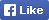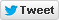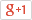Computer/Electronics Questions and Answers
C, C++, VC++, COM/DCOM, DLL and moreLoginSign upHome Electronics Computer Questions Member's Area News Site Map About

# Write a program to find the sum of the following series:

## Source Code

 1 #include 2 #include 3 int main (int argc, char *argv[]) 4 { 5 clrscr(); 6 int i=0,r=0,s=0,c=1; 7 printf("\n Enter the range:"); 8 scanf("%d",&r); 9 for(i=1;i<=r;i++) 10 { 11 s+=c; 12 c+=5; 13 } 14 printf("\n The sum of %d numbers of the series is %d",r,s); 15 getch(); 16 }

Find More from our code collection
Armstrong number, binary number to a decimal number, bubble sort, decimal number to binary number, factorial of the given number factors, fibonacci numbers, HCF and LCM, matrix, mergesort, salary of the employee. palindrome, quadratic equation, star patterns, series etc. and much more...Return to Example Source Code